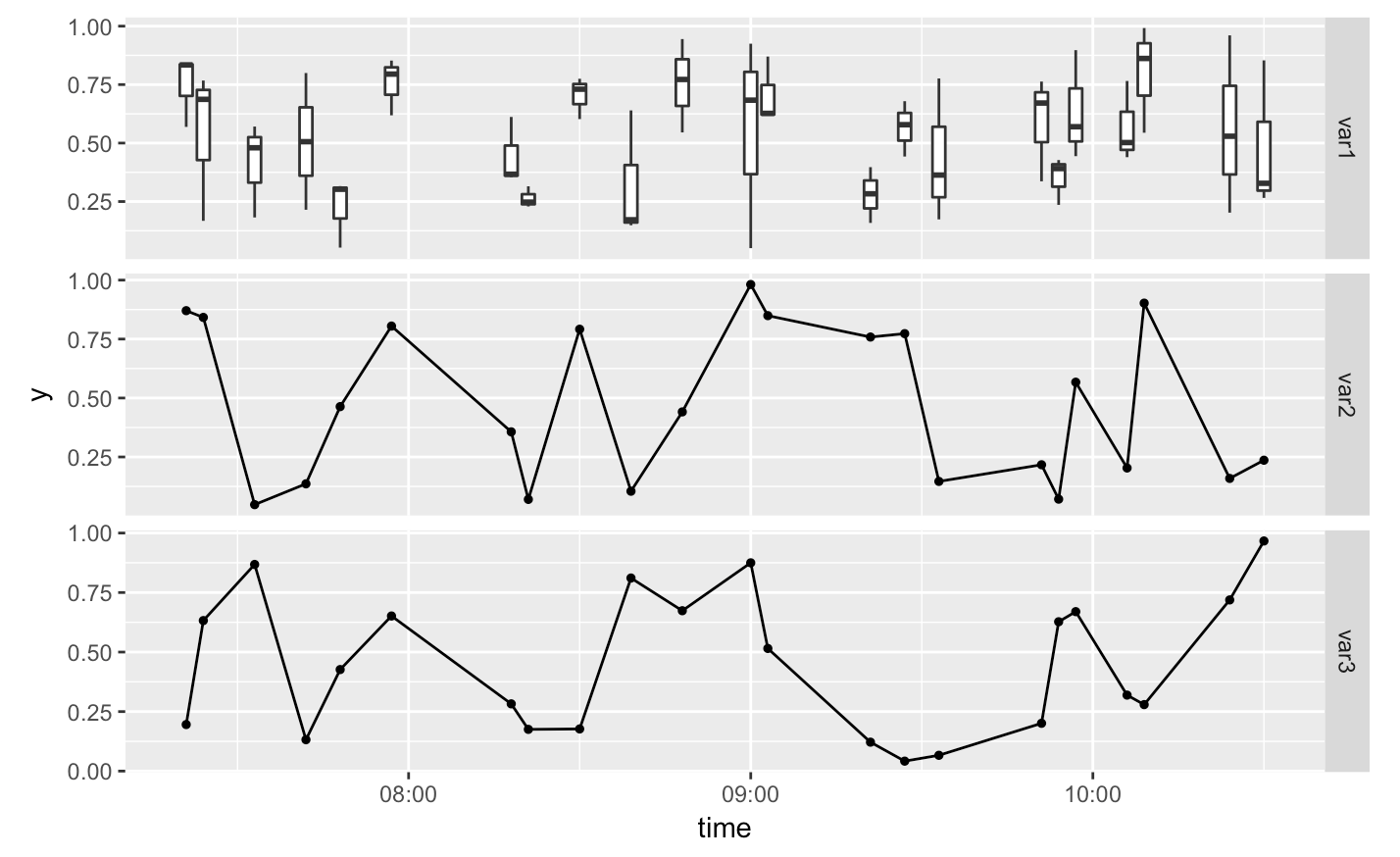# How to combine point and boxplots in timeline charts with ggplot2 facets

In a recent project, I was looking to plot data from different variables along the same time axis. The difficulty was, that some of these variables I wanted to have as point plots, while others I wanted as box-plots. Because I work with the tidyverse, I wanted to produce these plots with ggplot2. Faceting was the obvious first step but it took me quite a while to figure out how to best combine facets with point plots (where I have one value per time point) with and box-plots (where I have multiple values per time point).#### Dr. Shirin Elsinghorst

Biologist turned Bioinformatician turned Data Scientist

Data Scientist

Münster, Germany